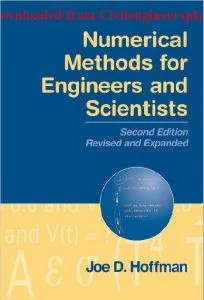# Numerical Methods for Engineers and Scientists

Second Edition Revised and Expanded By Joe D. HoffmanContents of Numerical Methods for Engineers and Scientists

Preface
Chapter 0.
Introduction
Objectives and Approach
Organization of the Book
Examples
Programs
Problems
Significant Digits, Precision, Accuracy, Errors, and NumbeRr epresentation
Software Packages and Libraries
The Taylor Series and the Taylor Polynomial
Part I
Basic Tools of Numerical Analysis
Systems of Linear Algebraic Equations
Eigenproblems
Roots of Nonlinear Equations
Polynomial Approximation and Interpolation
Numerical Differentiation and Difference Formulas
Numerical Integration
Summary
Chapter 1. Systems of Linear Algebraic Equations
1.1. Introduction
1.2. Properties of Matrices and Determinants
1.3. Direct Elimination Methods
1.4. LU Factorization
1.5. Tridiagonal Systems of Equations
1.6. Pitfalls of Elimination Methods
1.7. Iterative Methods
1.8. Programs
1.9. Summary
Exercise Problems
Chapter 2. Eigenproblems
2.1. Introduction
2.2. Mathematical Characteristics of Eigenproblems
2.3. The Power Method
2.4. The Direct Method
2.5. The QR Method
2.6. Eigenvectors
2.7. Other Methods
2.8. Programs
2.9. Summary ,
Exercise Problem
Chapter 3. Nonlinear Equations
3.1. Introduction
3.2. General Features of Root Finding
3.3. Closed Domain (Bracketing) Methods
3.4. Open Domain Methods
3.5. Polynomials
3.6. Pitfalls of Root Finding Methods and Other Methods of Root Finding
3.7. Systems of Nonlinear Equations
3.8. Programs
3.9. Summary
Exercise Problem.
Chapter 4. Polynomial Approximation and Interpolation
4.1. Introduction
4.2. Properties of Polynomials
4.3. Direct Fit Polynomials
4.4. Lagrange Polynomials
4.5. Divided Difference Tables and Divided Difference Polynomials
4.6. Difference Tables and Difference Polynomials
4.7. Inverse Interpolation
4.8. Multivariate Approximation
4.9. Cubic Splines
4.10. Least Squares Approximation
4.11. Programs
4.12. Summary
Exercise Problems
Chapter 5. Numerical Differentiation and Difference Formulas
5.1. Introduction
5.2. Unequally Spaced Data
5.3. Equally Spaced Data
5.4. Taylor Series Approach
5.5. Difference Formulas
5.6. Error Estimation and Extrapolation
5.7. Programs
5.8. Summary
Exercise Problems
Chapter 6. Numerical Integration
6.1. Introduction
6.2. Direct Fit Polynomials
6.3. Newton-Cotes Formulas
6.4. Extrapolation and RombergI ntegration
6.7. Multiple Integrals
6.8. Programs
6.9. Summary
Exercise Problems
Part II.
Ordinary Differential Equations
Introduction
General Features of Ordinary Differential Equations
Classification of Ordinary Differential Equations
Classification of Physical Problems
Initial-Value Ordinary Differential Equations
Boundary-ValueO rdinary Differential Equations
Summary
Chapter 7.
One-DimensionaIln itial-Value Ordinary Differential Equations
Introduction
General Features of Initial-Value ODEs
The Taylor Series Method
The Finite Difference Method
The First-Order Euler Methods
Consistency, Order, Stability, and Convergence
Single-Point Methods
Extrapolation Methods
Multipoint Methods
Summary of Methods and Results
Nonlinear Implicit Finite Difference Equations
Higher-Order Ordinary Differential Equations
Systems of First-Order Ordinary Differential Equations
Stiff Ordinary Differential Equations
Programs
Summary
Exercise Problems
Chapter 8.
One-Dimensional Boundary-Value Ordinary Differential Equations
Introduction
General Features of Boundary-Value ODEs
The Shooting (initial-Value) Method
The Equilibrium (Boundary-Value) Method
Derivative (and Other) Boundary Conditions
Higher-Order Equilibrium Methods
The Equilibrium Method for Nonlinear Boundary-Value Problems
The Equilibrium Method on Nonuniform Grids
Eigenproblems
Programs
Summary
Exercise Problems
Part III.
Partial Differential Equations
Introduction
General Features of Partial Differential Equations
Classification of Partial Differential Equations
Classification of Physical Problems
Elliptic Partial Differential Equations
Parabolic Partial Differential Equations
Hyperbolic Partial Differential Equations
The Convection-Diffusion Equation
Initial Values and Boundary Conditions
Well-Posed Problems
Summary
Chapter 9.
Elliptic Partial Differential Equations
Introduction
General Features of Elliptic PDEs
The Finite Difference Method
Finite Difference Solution of the Laplace Equation
Consistency, Order, and Convergence
Iterative Methods of Solution
Derivative Boundary Conditions
Finite Difference Solution of the Poisson Equation
Higher-Order Methods
Nonrectangular Domains
Nonlinear Equations and Three-Dimensional Problems
The Control Volume Method
Programs
Summary
Exercise Problems
Chapter 10.
Parabolic Partial Differential Equations
Introduction
General Features of Parabolic PDEs
The Finite Difference Method
The Forward-Time Centered-Space (FTCS) Method
Consistency, Order, Stability, and Convergence
The Richardson and DuFort-Frankel Methods
Implicit Methods
Derivative Boundary Conditions
Nonlinear Equations and Multidimensional Problems
The Convection-Diffusion Equation
Asymptotic Steady State Solution to Propagation Problems
Programs
Summary
Exercise Problems
Chapter 11.
Hyperbolic Partial Differential Equations
Introduction
General Features of Hyperbolic PDEs
The Finite Difference Method
The Forward-Time Centered-Space (FTCS) Method and the Lax Method
Lax-Wendroff Type Methods
Upwind Methods
The Backward-Time Centered-Space (BTCS) Method
Nonlinear Equations and Multidimensional Problems
The Wave Equation
Programs
Summary
Exercise Problems
Chapter 12
Finite Element Method
Introduction
The Rayleigh-Ritz, Collocation, and Galerkin Methods
The Finite Element Method for Boundary Value Problems
The Finite Element Method for the Laplace (Poisson) Equation
The Finite Element Method for the Diffusion Equation
Programs
Summary
Exercise Problems
References